# 什么是模糊字符串匹配？

Fuzzy（模糊）字符串匹配像一个老板。它作为一个简单易用的包使用Levenshtein Distance来计算中序列之间的差异。

# 要求

• Python2.7或更高版本
• 依赖于优秀的difflib Python库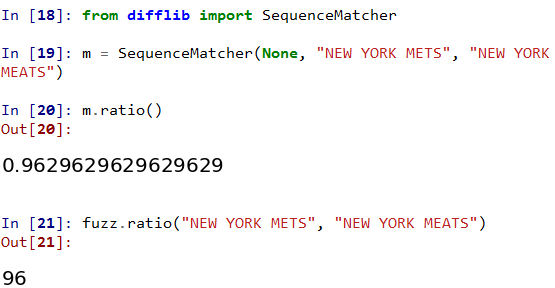• Python-Levenshtein（可选，在字符串匹配中提供4-10的加速，但在某些情况下可能会导致不同的结果）

# 安装

``pip install fuzzywuzzy``

``pip install fuzzywuzzy [speedup]``

``pip install git+git://github.com/seatgeek/fuzzywuzzy.git@0.17.0#egg=fuzzywuzzy``

``````git clone git://github.com/seatgeek/fuzzywuzzy.git fuzzywuzzy
cd fuzzywuzzy
python setup.py install``````

# 使用方法

``````>>> from fuzzywuzzy import fuzz
>>> from fuzzywuzzy import process``````

# 四种模糊匹配方法

1、ratio()——使用纯Levenshtein Distance进行匹配。

2、partial_ratio()——基于最佳的子串（substrings）进行匹配

3、token_set_ratio——对字符串进行标记（tokenizes）并在匹配之前按字母顺序对它们进行排序

4、token_set_ratio——对字符串进行标记（tokenizes）并比较交集和余数

### 1、当比较字符串因标点符号而不同的情况：

``fuzz.ratio("test is fuzzywuzzy","test is fuzzywuzzy") #抽取匹配``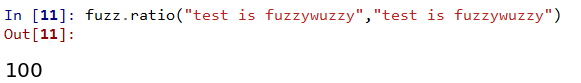`` fuzz.ratio("test is fuzzywuzzy","test is fuzzywuzzy..") #有标点``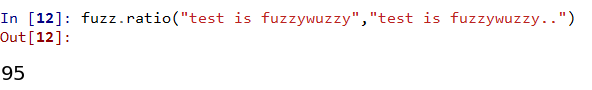``fuzz.partial_ratio("test is fuzzywuzzy","test is fuzzywuzzy..") #有标点``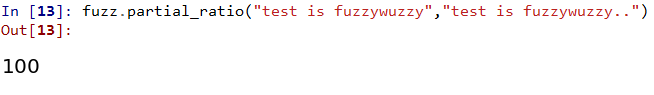token_sort_ratio方法涉及对有问题的字符串进行标记，按字母顺序对标记进行排序，然后将它们连接回字符串。例如：

``"new york mets vs atlanta braves"   →→  "atlanta braves mets new vs york"``
``fuzz.token_sort_ratio("test is fuzzywuzzy","test is fuzzywuzzy!!") #标点``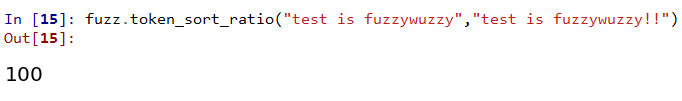token_set_ratio方法类似，但更灵活一点。在这里，我们对两个字符串进行标记，但不是立即进行排序和比较，而是将标记分为两组：交集和余数。我们使用这些集合来构建比较字符串。

``````s1 = "mariners vs angels"
s2 = "los angeles angels of anaheim at seattle mariners"

#使用token_sort_ratio方法没有用，因为第二个（更长）字符串有太多的额外token与排序交错。我们最终会比较：
t1 = "angels mariners vs"
t2 = "anaheim angeles angels los mariners of seattle vs"
#不是很有用。相反，set方法允许我们检测“angels”和“mariners ”对两个字符串都是通用的，并将它们分开#（设置交集）。现在我们构造并比较以下形式的字符串:
t0 = [SORTED_INTERSECTION]
t1 = [SORTED_INTERSECTION] + [SORTED_REST_OF_STRING1]
t2 = [SORTED_INTERSECTION] + [SORTED_REST_OF_STRING2]
# 然后比较每一对。
'''

'''
t0 = "angels mariners"
t1 = "angels mariners vs"
t2 = "angels mariners anaheim angeles at los of seattle"
fuzz.ratio(t0, t1) ⇒ 90
fuzz.ratio(t0, t2) ⇒ 46
fuzz.ratio(t1, t2) ⇒ 50
fuzz.token_set_ratio("mariners vs angels", "los angeles angels of anaheim at seattle mariners") ⇒ 90
'''

'''

fuzz.token_set_ratio("Sirhan, Sirhan", "Sirhan") ⇒ 100``````
``fuzz.token_set_ratio("test is fuzzywuzzy","test is fuzzywuzzy!!")``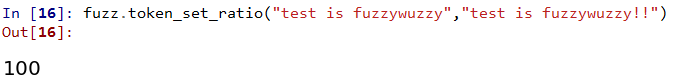### 2、比较字符串有不同的情况：

``fuzz.ratio("Test is Fuzzywuzzy","test is fuzzywuzzy") #ratio - 大小写``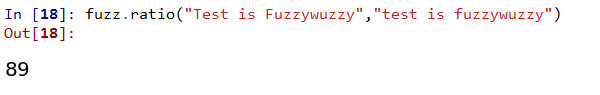`` fuzz.partial_ratio("Test is Fuzzywuzzy","test is fuzzywuzzy")#patial_ratio - 大小写``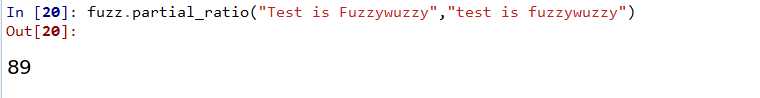``````fuzz.token_sort_ratio("Test is Fuzzywuzzy","test is fuzzywuzzy") #token_sort_ratio -大小写

``````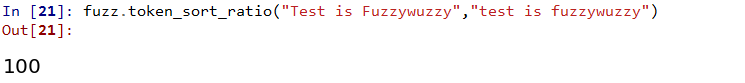``````fuzz.token_set_ratio("Test is Fuzzywuzzy","test is fuzzywuzzy") #token_set_ratio-大小写
``````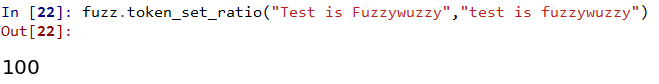### 3、比较字符串顺序不同的情况：

``fuzz.ratio("Test is Fuzzywuzzy","fuzzywuzzy is test ")``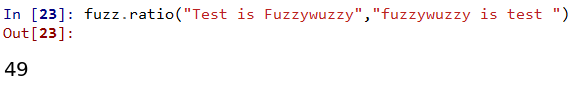``fuzz.partial_ratio("Test is Fuzzywuzzy","fuzzywuzzy is test ")``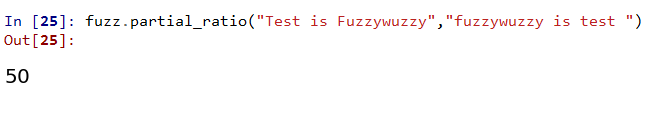``fuzz.token_set_ratio("Test is Fuzzywuzzy","fuzzywuzzy is test ")``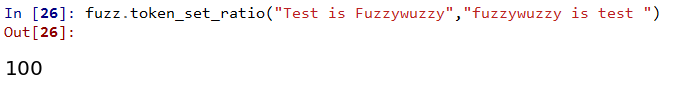`` fuzz.token_sort_ratio("Test is Fuzzywuzzy","fuzzywuzzy is test ")``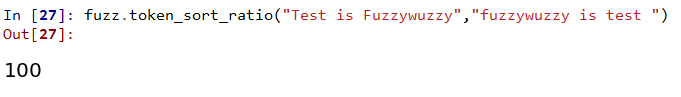### 4、比较字符串是子集

``fuzz.ratio("Test is Fuzzywuzzy","fuzzywuzzy ")``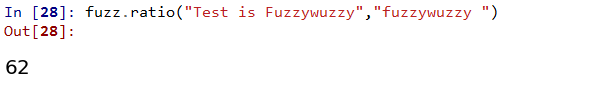`` fuzz.partial_ratio("Test is Fuzzywuzzy","fuzzywuzzy ")``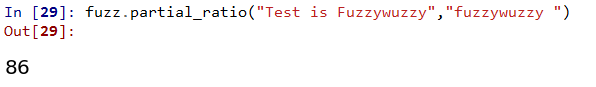``fuzz.token_sort_ratio("Test is Fuzzywuzzy","fuzzywuzzy ")``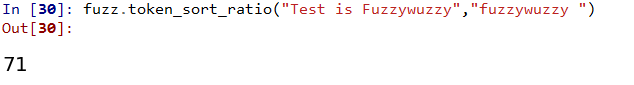``fuzz.token_set_ratio("Test is Fuzzywuzzy","fuzzywuzzy ")``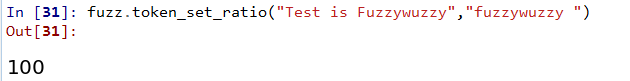## 与选择列表进行比较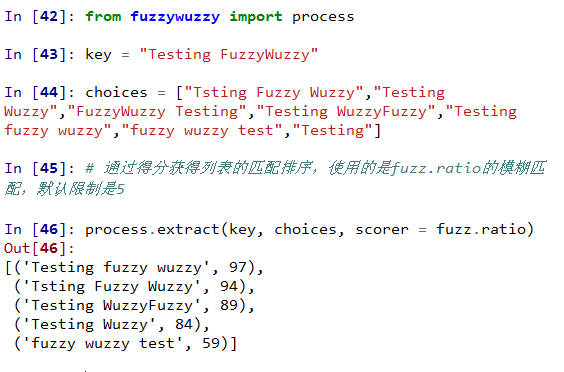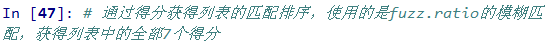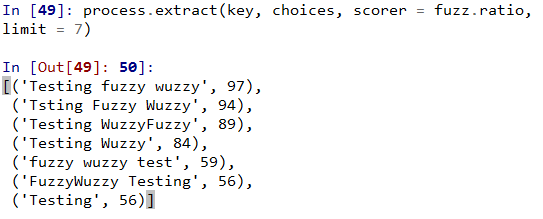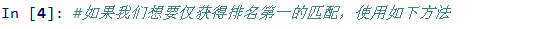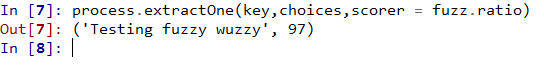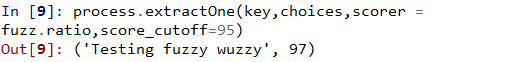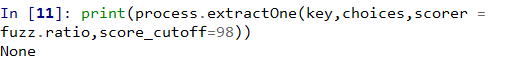## 将FuzzyMatch应用于整个数据集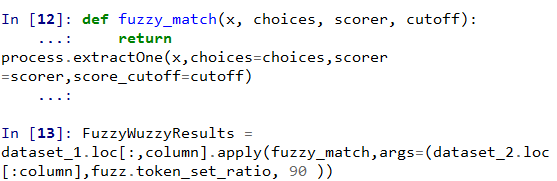## 更多模糊匹配用例：

• 匹配客户跟踪所有客户购买以识别购买行为
• 匹配客户地址以根据位置细分客户
• 查找搜索关键字的近似匹配项
• 匹配文件路径
• 用于拼写检查
• 检测剽窃（文本重用）
• 匹配DNA序列
• 用于垃圾邮件过滤

GitHub中FuzzyWuzzy

FuzzyWuzzy：Python中的模糊字符串匹配

Python中的模糊字符串匹配

THE END